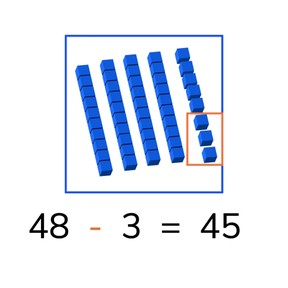Subtraction to 50 without crossing ten with subtrahends <10

# Subtraction to 50 without crossing ten with subtrahends <10

I can subtract numbers to 10 from numbers to 50 without crossing ten.

No account needed.8,000 schools use Gynzy92,000 teachers use Gynzy1,600,000 students use Gynzy

## General

Students learn to subtract numbers to 10 from numbers to 50 without regrouping tens.

2.NBT.B.5

## Relevance

It is important to be able to subtract numbers to 50 so you can determine how many you have left.

## Introduction

Have students practice numbers to 50 by asking students to come to the front of the class and dragging the number to where it belongs on the number line. Then as a class solve the subtraction problem on the number line to 30.

## Development

Start by discussing the importance of subtraction to 50. This learning goal is explained in three different methods, visually, in the abstract, and in story form. You can use the blue menu in the bottom right to select the method that is best suited to your classroom needs. Otherwise start at the first which is the visual method. Explain how to subtract using the blocks on the interactive whiteboard. Then have students practice a set of visual subtraction problems on their own. Next, explain how to subtract in the abstract, so without visual support. Tell students that it can be helpful to imagine a number line in their heads as they solve these subtraction problems. Then have students practice a set of abstract subtraction problems. Finally discuss the steps required to solve a story problem and do a story problem as s class. Then ask students to solve a story problem in pairs. Each method has explanation by the teacher, practice problems to do as a class, and then practice problems for the students to do to check their understanding.

Check that students are able to subtract numbers to 10 from numbers to 50 by asking the following questions:
- Why is it useful to be able to subtract to 50?
- What do you do with the second number (minuend) in a subtraction problem?

## Guided practice

Students are first given a subtraction problem with visual support, then one in the abstract, and finally a story problem.

## Closing

As a class, do a visual, abstract, and story subtraction problem.

## Teaching tips

Students who have difficulty with this goal can be supported by making use of manipulatives, like blocks, to use when solving the subtraction problems.

## Instruction materials

Optional: blocks or other manipulatives

### The online teaching platform for interactive whiteboards and displays in schools

• Save time building lessons

• Manage the classroom more efficiently

• Increase student engagement